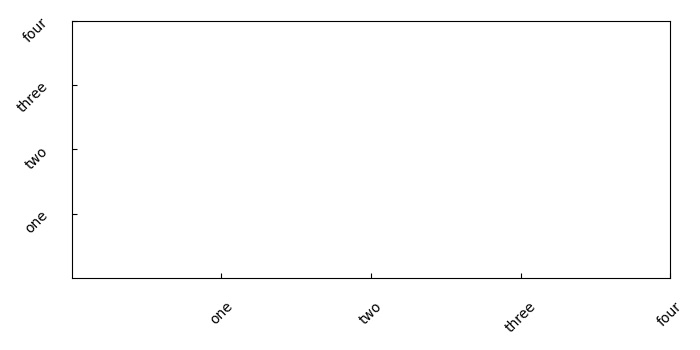# How to rotate tick labels in a subplot in Matplotlib?

To rotate tick labels in a subplot, we can use set_xticklabels() or set_yticklabels() with rotation argument in the method.

• Create a list of numbers (x) that can be used to tick the axes.

• Get the axis using subplot() that helps to add a subplot to the current figure.

• Set ticks on the X and Y axes using set_xticks and set_yticks methods, respectively, and the list x (from step 1).

• Set tick labels with label lists (["one", "two", "three", "four"]) and rotation=45 using set_xticklabels() and set_yticklabels().

• To add space between axes and tick labels, we can use tick_params() method with pad argument that helps to add space. The argument direction(in) helps to put the ticks inside the axes. And, apply axis(both) parameters on both the axes.

• To show the figure, use plt.show() method.

## Example

import matplotlib.pyplot as plt
plt.rcParams["figure.figsize"] = [7.00, 3.50]
plt.rcParams["figure.autolayout"] = True
x = [1, 2, 3, 4]
ax1 = plt.subplot()
ax1.set_xticks(x)
ax1.set_yticks(x)
ax1.set_xticklabels(["one", "two", "three", "four"], rotation=45)
ax1.set_yticklabels(["one", "two", "three", "four"], rotation=45)
plt.show()